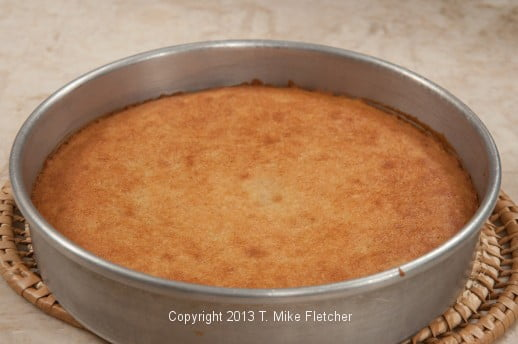# Pan Size Conversions

BY HELEN S. FLETCHER, ON
ALL PHOTOS BY PASTRIES LIKE A PRO UNLESS OTHERWISE NOTED.Pan size conversion is one of the most confusing elements of baking whether it is substituting square pans for round pans or for the same shape pans in smaller or larger size.

The easiest way to convert the amount of batter is to use square inches so long as the depth of the pans are the same.

You will notice that square pans of the same size cannot be substituted for round ones if you wish the layers to be the same depth.  When converting from round to square pans it takes approximately 1/3 more batter for the square ones.

If you are going from a 2” to a 3” pan of the same shape, increase the amount by 1/3.

When you are changing the size, the temperature of the oven remains the same but the time may need to be adjusted up or down depending upon the thickness of the layers.

In my recipes, I give the weights of the finished batter as well as how much should go in each pan.  For instance, if a base layer weighs 750 grams or 26 ounces, it can easily be divided into different size layers.   This allows you to use a base recipe with different fillings requiring a different number of layers without recalculating each time, making pan size conversions very easy.  Using the 750 grams you could make:

2 – 9” layers at 375 grams or 13 ounces each
3 – 9” layers at 250 grams each or 9 ounces each
4 – 9” layers at 185 grams each or 6 1/2 ounces each

CALCULATING SQUARE INCHES FOR SQUARE OR RECTANGULAR PANS:    For the square pan, multiply two sides of the pan.  For instance for an 8 inch square pan, multiply 8×8 = 64 square inches.  For a rectangle, simply take the measurements of the long side and the short side of a rectangular one and multiply  them, i.e. 9×13=117 square inches.

8” = 64 square inches
9” = 81 square inches
10” =100 square inches
12” =144 square inches
14” = 195 square inches
16” = 256 square inches
18” = 325 square inches
20” = 400 square inches
9”x13” pan – 9×13” = 117 square inches
Quarter sheet pan – 9×13″ =117 square inches
Half sheet pan – 18×13″ = 234 square inches
Full sheet pan – 18×26″ = 468 square inches

From this you can see that you can substitute two 8” pans for the 9”x13” one as  2×64 = 128 square inches which is close enough.

CALCULATING SQUARE INCHES FOR ROUND PANS is a little more complicated.

The formula is:  Divide the diameter of the pan in half to obtain the radius.  The radius is simply 1/2 the size of the pan.  Multiply that number by itself and then multiply that number by pi which is 3.14.

For instance, the radius of a 9” pan is 4.5 inches.

4.5×4.5 = 20.25 inches.
20.25×3.14 = 63.58 which would round off to 64 square inches.

8” = 50 square inches
9” = 64 square inches
10” = 79 square inches
12” = 114 square inches
14” = 154 square inches
16” = 201 square inches
18” =  254 square inches
20” = 314 square inches

You can see from these measurements, that the batter for a 9″ square pan would make one 10″ round one.  The numbers will not be exact, but as long as they are close they will be fine.

A SECOND WAY to determine pan size is to measure the amount in a pan by cups. I have never used this method as weights are more exact and so much easier.

SaveSave

# Cake Pan Prep or How to Get a Flat Cake Layer

BY HELEN S. FLETCHER, ONOne of the most frustrating aspects of baking is to spend time making a scratch cake, putting it into the oven and waiting for it to bake – only to have layers that are humpy in the center.  Of course, they can be trimmed flat but why not bake them that way to begin with?  In a professional bakery, trimming takes time and wastes money.